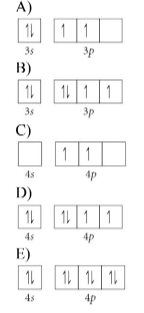# Problem: Choose the valence orbital diagram that represents the ground state of Se 2−.

###### FREE Expert Solution

We’re being asked to construct the orbital diagram for Se2–

For this problem, we need to do the following:

Step 1: Determine the electron configuration of the neutral element.

Step 2: Determine the electron configuration of the ion.

Step 3: Construct the orbital diagram for the ion.###### Problem Details

Choose the valence orbital diagram that represents the ground state of Se 2−.